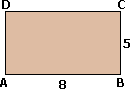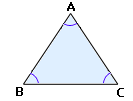## Definition Of Perimeter

Perimeter is the distance around a polygon.

### Examples of Perimeter

The perimeter of the rectangle shown is AB + BC + CD + DA = 8 + 5 + 8 + 5 = 26 units.
Perimeter of a rectangle can also be found using the formula 2(l + w), where l is the length and w is the width.

### Solved Example on Perimeter

#### Ques: ABC is an equilateral triangle and the perimeter of triangle ABC is 12 in. Find the measure of each side.A. 36 in.
B. 4 in.
C. 12 in.
D. 6 in.